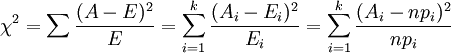• 基于Matlab实现的卡方检验源代码，欢迎下载，学习交流 1 基于Matlab实现的卡方检验源代码，欢迎下载，学习交流 1
• 基于Matlab实现的卡方检验源代码，欢迎下载，学习交流。
•matlab
• CHISQUARECONT 将表示 2x2 列联表的 2x2 矩阵作为输入，并且计算获得观察到的和每个更极端的表的概率基于卡方分布的皮尔逊卡方检验。 这当预期频率的总数增加时，卡方检验可能变得不可靠（列联表中的单元格值）不够...matlab
• H = CHI2TEST2(X) 执行卡方二样本检验两个样本的相似性，在零假设下两个样本来自相同（未指定）的分布。 X1 和 X2 是两个包含离散（分类）数据的向量。 CHI2TEST2 将所有唯一值视为单独的“bins”进行测试目的。 X1...matlab
• 同质性和独立性的卡方检验。 计算 I x J 的 P 值 - 表行/列独立性。 参考：DeltaProt 工具箱位于http://services.cbu.uib.no/software/deltaprot/ 输入： X：观察到的频率单元的数据矩阵（I x J -table）。 方法：'...matlab
• 卡方检验Matlab

万次阅读 2015-10-10 23:12:14
• chi2test（数据，numOfInterval） 数据：输入随机数numOfInterval：间隔数要求： n/k >= 5 k >= 100matlab
• 用法：[p, Q]= chi2test(x) 卡方检验。 给定大量样本，该函数检验样本是独立的。 如果 Q > chi2(p, nu)，则假设被拒绝。 每列代表一个变量，每行代表一个样本。 如果您发现任何错误，请告诉我： . 参数： x 绝对...matlab
• clc;clear;close all; K=[1.1 2.2 3.3 4.4]; P=imread('lenna.bmp'); P1=rgb2gray(P); P_R=P(:,:,1);P_G=P(:,:,2);P_B=P(:,:,3); imhist(uint8(P(:,:,1))); P1=double(P1); iptsetpref('imshowborder',...figure(3
clc;clear;close all;
K=[1.1 2.2 3.3 4.4];
P1=rgb2gray(P);

P_R=P(:,:,1);P_G=P(:,:,2);P_B=P(:,:,3);
imhist(uint8(P(:,:,1)));

P1=double(P1);
iptsetpref('imshowborder','tight');

figure(1);imshow(P1);
% figure(2);imshow(P2);figure(3);imshow(P3);
% figure(1);imshow(uint8(P(:,:,1)));figure(2);imshow(uint8(P_G));figure(3);imshow(P_B);
% C1=TpEncrypt(P1,K);C2=TpEncrypt(P2,K);C3=TpEncrypt(P3,K);
% figure(4);imshow(C1);figure(5);imshow(C2);figure(6);imshow(C3);

% P1=double(P1);P2=double(P2);P3=double(P3);
figure(7);
hist(P1(:),256);set(gca,'fontsize',18);
% hist(P_R,256);set(gca,'fontsize',18);
% figure(8);hist(P2(:),256);set(gca,'fontsize',18);
% figure(9);hist(P3(:),256);set(gca,'fontsize',18);
% C1=double(C1);C2=double(C2);C3=double(C3);
% figure(10);hist(C1(:),256);set(gca,'fontsize',18);
% figure(11);hist(C2(:),256);set(gca,'fontsize',18);
% figure(12);hist(C3(:),256);set(gca,'fontsize',18);

[M,N]=size(P1);g=M*N/256;
fp1=hist(P1(:),256);
%  fp2=hist(P2(:),256);fp3=hist(P3(:),256);
% fp1=hist(P_R,256);
% fp2=hist(P2(:),256);fp3=hist(P3(:),256);
chai1=sum((fp1-g).^2)/g;
% chai2=sum((fp2-g).^2)/g;chai3=sum((fp3-g).^2)/g;
% fc1=hist(C1(:),256);fc2=hist(C2(:),256);fc3=hist(C3(:),256);
% chai4=sum((fc1-g).^2)/g;chai5=sum((fc2-g).^2)/g;chai6=sum((fc3-g).^2)/g;

展开全文• function [p, Q]= chi2test(x)% Usage: [p, Q]= chi2test(x)%% The chi-squared test.%% Given a number of samples this function tests the hypothesis that the samples are% independent. If Q >...

function [p, Q]= chi2test(x)

% Usage: [p, Q]= chi2test(x)

%

% The chi-squared test.

%

% Given a number of samples this function tests the hypothesis that the samples are

% independent. If Q > chi2(p, nu), the hypothesis is rejected.

%

% Each column represents a variables, each row a sample.

%

% If you find any errors, please let me know: .

%

% ARGUMENTS:

% x     Absolut numbers.

% p     The prob ability value, calculated from Q.

% Q     The resulting Q-value.

%

% EXAMPLE 1

% In region A, 324 of 556 cows were red, whereas in region B 98 of 260 were red.

% [p, Q]= chi2test([324, 556-324; 98, 260-98])

% p=

%    4.2073e-08

% Q=

%    30.0515

% With an error risk of about 4e-08, we can claim that the samples are independent.

%

% EXAMPLE 2

% Throw two different dices to see if they have the same probability of 1 (and 2, 3, 4, 5, 6).

% We don't check if they are symetrical, only if the both behave in the same way.

% [p,Q] = chi2test([15,10; 7,11; 9,7; 20,15; 26,21; 19,16])

% p=

%    0.8200

% Q =

%    2.2059

% The dices don't significantly behave differently. That is, they seem to behave in the same way.

%

% HISTORY:    v.1.0, first working version, 2007-08-30.

%

% COPYRIGHT:  (c) 2007 Peder Axensten. Use at own risk.

% KEYWORDS:   chi-squared test, chi-squared, chi2, test

%%%%%%%%%%%%%%%%%%%%%%%%%%%%%%%%%%%%%%%%%%%%%%%%%%%%%%%%%%%%%%%%%%%%%%%%%%%%%

% Check the arguments.

if(nargin ~= 1),                        error('One and only one argument required!');                                        end

if(ndims(x) ~= 2),                        error('The argument (x) must be a 2d matrix!');                                        end

if(any(size(x) == 1)),                error('The argument (x) must be a 2d matrix!');                                        end

if(any(~isreal(x))),                error('All values of the argument (x) must be real values!');        end

% Calculate Q = sum( (a-np*)^2/(np*(1-p*)) )

s=                size(x, 1);

r=                size(x, 2);

np=                sum(x, 2)/sum(sum(x)) * sum(x);                % p=sum(x, 2)/sum(sum(x)) and n=sum(x)

Q=                sum(sum((x-np).^2./(np)));

% Calculate cdf of chi-squared to Q. Degrees of freedom, v, is (r-1)*(s-1).

p=                1 - gammainc(Q/2, (r-1)*(s-1)/2);

end

展开全文• 2.卡方检验的应用条件四格表:1).随机样本数据；2).卡方检验的理论频数不能太小。两个独立样本比较可以分以下3种情况：1.所有的理论数T≥5并且总样本量n≥40，用Pearson卡方进行检验。2.如果理论数T但T≥1，并且n≥40...

拟合性检验是用于分析实际次数与理论次数是否相同，适用于单个因素分类的计数数据。独立性检验用于分析各有多项分类的两个或两个以上的因素之间是否有关联或是否独立的问题。

2.卡方检验的应用条件

四格表:

1).随机样本数据；

2).卡方检验的理论频数不能太小。

两个独立样本比较可以分以下3种情况：

1.所有的理论数T≥5并且总样本量n≥40，用Pearson卡方进行检验。

2.如果理论数T＜5但T≥1，并且n≥40，用连续性校正的卡方进行检验。

3.如果有理论数T＜1或n＜40，则用Fisher’s检验。

R×C表卡方检验应用条件：

1.R×C表中理论数小于5的格子不能超过1/5；

2.不能有小于1的理论数。我的实验中也不符合R×C表的卡方检验。可以通过增加样本数、列合并来实现。

3. Matlab实现

Matlab中有两个chi-square test相关函数：

4. Excel实现

Pearson χ2统计量(i=1,2…)

利用Excel提供的CHIINV函数求临界值，如 =CHIINV(0.05, 5)

5. SPSS实现

类似Matlab，SPSS中也有两种方法：

分析——描述统计——交叉表

【注】a.使用第二种方法“交叉表”前，要先进行加权

b. 多于2行2列的R*C表，在交叉表——精确选项中应选Fisher精确检验

连续性校正卡方检验：仅适用于四格表资料，在n>40，所有期望频数均大于1，只有1/5单元格的期望频数大于1小于5时；

Fisher精确概率法：在样本含量<40或有格子的期望频数<1的列联表，应该采用该法；

展开全文• N 指定检验的等概率类区间数。 ALPHA 是用于找到临界卡方值的置信水平参数。 DIST 是一个包含我们正在测试的概率分布的字符串。 请参阅 staitsctics 工具箱以了解支持的发行版 - 'exp'、'gam'、'unif' 是其中的...matlab
• chi2test，matlab里的卡方检验

千次阅读 2020-08-23 21:24:52
来源是https://ww2.mathworks.cn/matlabcentral/fileexchange，完整代码如下： function [p, Q]= chi2test(x) % Usage: [p, Q]= chi2test(x) % % The chi-squared test. % % Given a number of samples this ...matlab
• 卡方检验是专用于解决计数数据统计分析的假设检验法。本章主要介绍卡方检验的两个应用：拟合性检验和独立性检验。拟合性检验是用于分析实际次数与理论次数是否相同，适用于单个因素分类的计数数据。独立性检验用于...
• 基于： https : //stattrek.com/chi-square-test/homogeneity.aspx?tutorial= 对同质性的简单卡方检验，其中您有来自多个总体的单个分类变量。 X应该是一个数组，其中行是总体，列是类别。 输出p值和卡方统计量。matlab
• function [p, Q]= chi2test(x)% Usage: [p, Q]= chi2test(x)%% The chi-squared test.%% Given a number of samples this function tests the hypothesis that the samples are% independent. If Q >...
• 卡方检验（详解）

万次阅读 多人点赞 2018-08-16 10:57:47
卡方检验 卡方检验是一种用途很广的计数资料的假设检验方法。属于非参数检验，主要是比较两个及两个以上样本率（构成比）以及两个分类变量的关联性分析。根本思想在于比较理论频数和实际频数的吻合程度或者拟合优度...卡方检验 统计学
• 针对低成本惯性测量单元精度受载体机动影响大、先验知识难以准确获知的问题,提出一种采用卡方检验的模糊自适应无迹卡尔曼滤波组合导航算法.首先,根据惯性测量单元的基本情况构造系统噪声的粗略模型;然后,引入卡方...
• H=CHI2TEST(X,ALPHA) 执行 Pearson 卡方检验的特殊情况，以确定复合正态性 PDF 的原假设是否是关于具有所需显着性水平 ALPHA 的随机样本 X 的总体分布的合理假设。 H表示根据条件语句的MATLAB规则进行假设检验的...matlab
• 该函数在很大程度上依赖于 Matlab 的 Statistical Toolbox 来获取卡方值和 ktaub.m 函数。 这些测试将考虑到关系、缺失数据和每个时间索引的多次观察，因为它使用了最近更新的增强型 ktaub.m 函数。 有许多叙述...matlab
• h = chi2cont(x) 对 m×n 列联表 x 中的数据执行卡方检验。 零假设是列（“处理”）之间的行变量分布（“结果”）没有差异。 测试结果在 h 中返回。 h=1 表示在 5% 显着性水平拒绝原假设。 h=0 表示不能在 5% 拒绝原...matlab
• 皮尔逊卡方检验简记

千次阅读 2019-07-05 16:15:46
皮尔逊卡方检验简单应用： 1 分布服从某有限已知离散分布 自由度df=m-1， p-score分值越接近0表服从分布；接近1表不接受。 2 独立性检验 自由度df=(r-1)(c-1) p-score分值越接近0表拒绝独立。 3 分布...
• 计算拟合优度指标，包括残差平方和SSE、均方根误差RMSE检验卡方χ2检验、判定系数R2检验
• [h,p, chi2stat,df] = prop_test(X, N, 正确) 比较两个比例的简单卡方检验这是一个 alpha=0.05 的 2 边测试 输入： * X = 每个样本的成功次数向量（例如 [20 22]） * N = 每个样本的总计数向量（例如 [48 29]） *...matlab
• %chi2:卡方分布 %f:F分布 %weib:Weibull分布 %--------- %cdf:cumulative distribution function %pdf:probability density function %inv:分布函数反函数 %rdn:相应分布的随机数发生函数 %stat:相应分布的统计量...
• 卡方检验（chi square test）能够是一种假设性检验的方法，它能够检验两个分类变量之间是否是独立无关的。它通过观察实际值和理论值的偏差来确定原假设是否成立，它按照以下步骤来检验两个分类变量是否是独立的。 ...
• 针对具有遗传性疾病和性状的遗传位点分析问题，使用假设检验的方法，分别采用卡方检验、逻辑回归、SKAT 以及metaCCA 方法建立数学模型，使用MATLAB 和R 语言及其工具包进行编程，在合理的假设下，确定了与遗传性疾病......

卡方检验matlabmatlab 订阅